# Physical Chemistry : Reaction Equilibrium

## Example Questions

← Previous 1

### Example Question #1 : Equilibrium Constant And Reaction Quotient

Consider the following reaction.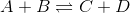A researcher adds equal volumes of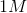substance A and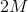substance B together. What is the ratio of concentration of C to concentration of D at equilibrium?

Cannot be determined from the given information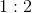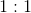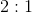Explanation:

To solve this question we need to use the definition of equilibrium constant. The equilibrium constant,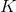, for this reaction is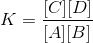For a givenvalue, the concentration of C and D would be the same. The stoichiometric coefficients of C and D are both 1; therefore, the amount of C and D produced would be the same and would depend on the limiting reagent. We do not know what the limiting reagent is (it could be A or B) and cannot determine the absolute concentrations of C and D; however, we can determine the relative concentrations. The concentration of C and D are the same and the ratio is 1:1.

### Example Question #1 : Reaction Equilibrium

The reaction quotient of a reaction is twice as much as its equilibrium constant. What can you conclude about this reaction?

Both forward and reverse reactions are occurring at a slower rate

The forward reaction is occurring at a faster rate

Both forward and reverse reactions are occurring at a faster rate

The reverse reaction is occurring at a faster rate

The forward reaction is occurring at a faster rate

Explanation:

The reaction quotient,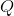, is calculated the same way as equilibrium constant; however, the concentrations used are derived from a nonequilbrium state. Consider the reaction below.If this reaction is NOT in equilibrium, then the reaction quotient is defined as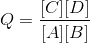If it’s at equilibrium then equilibrium constant is defined asNote that reaction quotient cannot be calculated when reaction is in equilibrium.

The question states that the reaction quotient is twice as much as the equilibrium constant. Since we can calculate reaction quotient, this reaction is not in equilbrium. The numerator foris higher than the numerator of(because reaction quotient is higher). There are more products created than reactants in the current nonequilbrium state and, therefore, the forward reaction is happening faster than the reverse reaction.

### Example Question #141 : Physical Chemistry

Which of the following is true about equilibrium?

I. Enzymes increase the equilibrium constant

II. Equilibrium constant is only dependent on temperature

III. At equilibrium, there are no new products produced

I and III

II only

I only

II and III

II only

Explanation:

Chemical equilibrium is the state during which the rate of forward reaction equals the rate of reverse reaction. Equilibrium properties of a reaction determine how much products is produced. It’s important to remember that enzymes alter the rate of a reaction; however, they DO NOT alter the equilibrium. This means that enzymes speed up a reaction; however, they do not increase the amount of products produced.

ONLY increasing or decreasing temperature will alter equilibrium constant. Other factors such as concentration, volume, and pressure do not change it. Changing these other factors might shift the reaction left or right to bring the reaction back to equilibrium; however, it does not change the equilibrium constant. This means that these other factors can change the individual concentration of reactants and products at equilibrium, but they will not change the ratio of products to reactants.

As mentioned, at equilibrium the rates of forward and reverse reactions are equal. This means that new products and reactants are constantly being produced; however, there is no net production of these molecules.

### Example Question #1 : Reaction Equilibrium

According to Le Chatelier’s principle, the reaction will shift to the right (towards the products) when the __________ is less than __________.

equilibrium constant . . . activation energy

reaction quotient . . . activation energy

reaction quotient . . . equilibrium constant

equilibrium constant . . . reaction quotient

reaction quotient . . . equilibrium constant

Explanation:

Le Chatelier’s principle states that a chemical system will respond to changes in the environment and maintain equilibrium by changing the direction of reaction. A reaction will shift to the right if the ratio of concentration of products to reactants goes down and will shift to the left if the ratio goes up. Reaction quotient,, characterizes the state of a reaction in a nonequilbrium state. It is calculated the same way as equilibrium constant.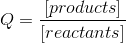The question is asking about a shift to the right. Reaction shifts to the right (and approaches equilbrium) when the nonequilbrium reaction has more reactants than products. This occurs when the ratio of products to reactants () is lower than the ratio of products to reactants at equilibrium ().

### Example Question #5 : Equilibrium Constant And Reaction Quotient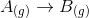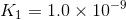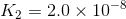What is the value of the equilibrium constant for the following reaction?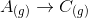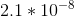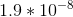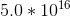There is not enough information to answer this question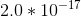Explanation:

Hess's Law allows us to combine the two given equation to create the equation that we need. Hess's Law also tells us that when combining equilibrium constants, the value is the product of K1 and K2

Remark: a common misconception in this type of problem is to find the sum of the values. This use of Hess's Law is only valid for thermodynamic values.

### Example Question #2 : Reaction Equilibrium

Ammonia is created using the Haber-Bosch process: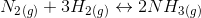A reaction vessel is used to combine the nitrogen and hydrogen gas. The reaction proceeds until the vessel is at equilibrium.

What would you predict to happen if the pressure of the vessel increases?

More ammonia would be created

Changing the pressure will not alter the equilibrium

More nitrogen gas would be created

The system will shift to the left

More ammonia would be created

Explanation:

According to Le Chatelier's principle, a change in pressure will cause a shift in order to counteract the pressure change. When the pressure of a vessel is increased, the side of the reaction with fewer gas molecules will be preferred in order to minimize contact between gas molecules. For this reaction, there are four gas molecules on the left side and two gas molecules on the right side. As a result, the products side will be preferred and more ammonia will be created.

### Example Question #3 : Reaction Equilibrium

Ammonia is created using the Haber-Bosch process: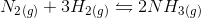A reaction vessel is used to combine the nitrogen and hydrogen gas until the vessel is at equilibrium.

What will happen to the system if ammonia is removed from the vessel?

The amount of hydrogen gas will increase

The equilibirum will shift to the right

The equilibrium will shift to the left

The equilibrium will not shift

The equilibirum will shift to the right

Explanation:

When a system is at equilibrium, it is possible to predict how a system will respond to sudden changes using Le Chatelier's principle. In this scenario, ammonia has been removed from the system. This will cause the reaction to produce more ammonia, and proceed to the right in order to reestablish equilibrium.

### Example Question #24 : Equilibrium And Kinetics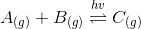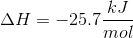Based on the given information, under what conditions should this reaction be carried out to promote the most formation of product?

High pressure, low temperature, under a lamp

Low pressure, high temperature, in the dark

The reaction will proceed as written regardless of conditions because it is spontaneous

Low temperature, low pressure, in a semi-lit area

High pressure, high temperature, in a vacuum

High pressure, low temperature, under a lamp

Explanation:

High pressure will favor the side of the reaction with fewer moles of gas, which in this case is the product side.

The reaction is exothermic, meaning that it generates heat. By removing heat from the reaction, we are constantly stressing the system towards the product side.

Finally, the reaction should occur under a lamp. This is due to the hv symbol above the double arrows, which signifies that light promotes the reaction. Note: had the hv symbol been below the double arrows, the reaction should have occurred in the dark.

### Example Question #1 : Reaction Equilibrium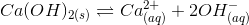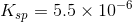A saturated aqueous solution of calcium hydroxide is prepared. Given the information above, how will adding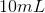of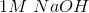affect the solubility and the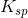of the solution?

The solubility will decrease; thewill remain unchanged.

The solubility will increase; thewill increase

The solubility will remain unchanged; thewill remain unchanged

The solubility will decrease; thewill decrease

The solubility will increase; thewill remain unchanged

The solubility will decrease; thewill remain unchanged.

Explanation:

Adding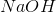contributes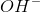ions into the solution, driving the reaction to the left, and decreasing the solubility of the calcium hydroxide. This is known as the common-ion effect. Theremains unchanged. This is due to the fact that only a change in temperature can bring about a change in an equilibrium constant.

### Example Question #1 : Acid Base Equilibrium And Titration

A 50mL solution of 0.2M hydrochloric acid is titrated with a 0.2M sodium hydroxide solution. What is the pH of the solution after 48mL of the sodium hydroxide solution has been added?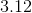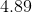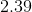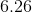Explanation:

Since a strong acid is being titrated with a strong base, we can simply subtract how many moles of base have been added from how many moles of acid were originally present.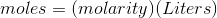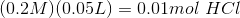Using the same equation, we found we have added 0.0096 moles of sodium hydroxide.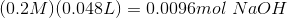Subtracting this amount from the hydrochloric acid leaves us with 0.0004 moles of acid.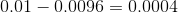Keep in mind that we must divide this molar amount by the new volume, after the base has been added.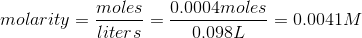Since this is a strong acid, we can simply take the negative log of this concentration, and are left with a pH of 2.39.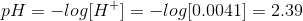← Previous 1

### All Physical Chemistry Resources